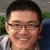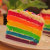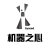Dan Jurafsky ，人文学科教授，斯坦福大学计算机科学教授兼语言学主席，研究自然语言处理及其在认知和社会科学中的应用。0+0+0+0+0+0+0+0+0+0+0+0+0+0+0+0+0+0+0+0+0+0+1+0+0+2+0+0+0+0+0+0+0+0+1+0+0+0+0+0+0+0+0+0+0+
Top